# NCERT Solutions for Class 6 Maths Chapter 7 Fractions Ex 7.2

NCERT Solutions for Class 6 Maths Chapter 7 Fractions Ex 7.2 are part of NCERT Solutions for Class 6 Maths. Here we have given NCERT Solutions for Class 6 Maths Chapter 7 Fractions Ex 7.2.

 Board CBSE Textbook NCERT Class Class 6 Subject Maths Chapter Chapter 7 Chapter Name Fractions Exercise Ex 7.2 Number of Questions Solved 3 Category NCERT Solutions

## NCERT Solutions for Class 6 Maths Chapter 7 Fractions Ex 7.2

Question 1.
Draw number lines and locate the points on them: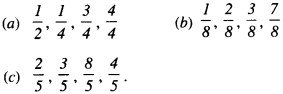Solution :
(a)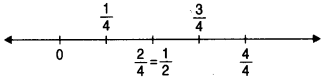(b)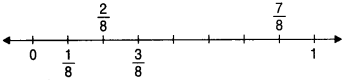(c)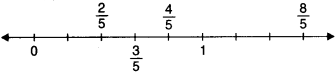Question 2.
Express the following as mixed fractions:
(a) $$\frac { 20 }{ 3 }$$
(b) $$\frac { 11 }{ 5 }$$
(c) $$\frac { 17 }{ 7 }$$
(d) $$\frac { 28 }{ 5 }$$
(e) $$\frac { 19 }{ 6 }$$
(f) $$\frac { 35 }{ 9 }$$.
Solution :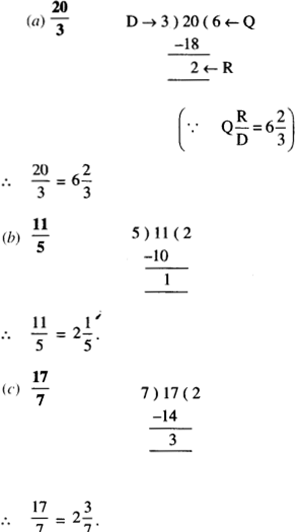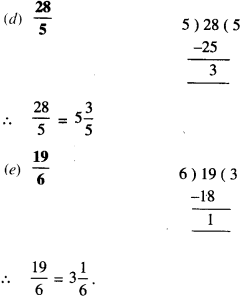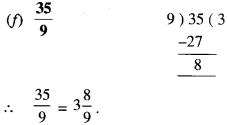Question 3.
Express the following as improper fractions: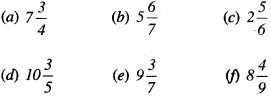Solution :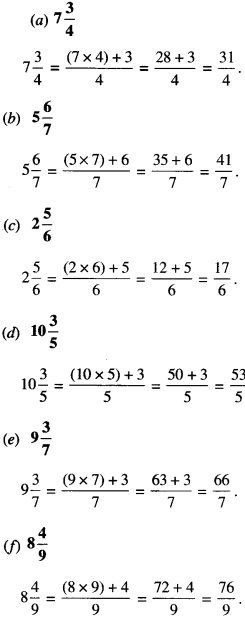We hope the NCERT Solutions for Class 6 Maths Chapter 7 Fractions Ex 7.2 help you. If you have any query regarding NCERT Solutions for Class 6 Maths Chapter 7 Fractions Ex 7.2, drop a comment below and we will get back to you at the earliest.